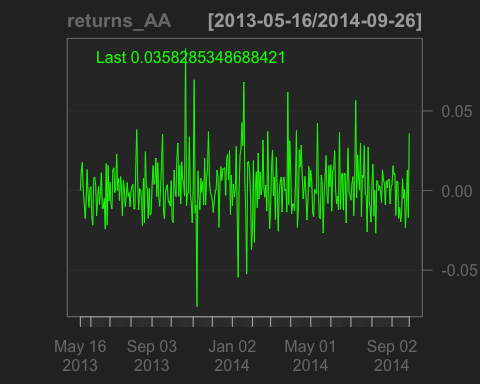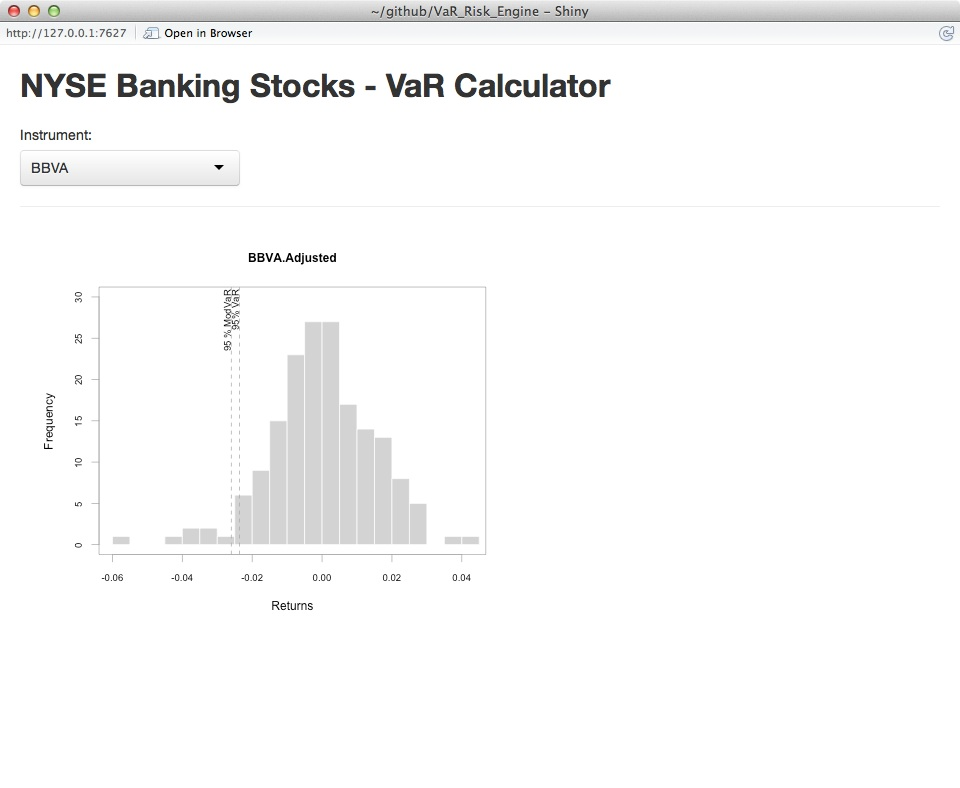## Calculating Value at Risk using R

### Tags: Big Data, Damian Spendel, Financial Services, Innovation, R, Value, VaR

#### 1

Introduction

My recent article focused on using R to perform some basic exploratory data analysis1.

The focus of this article will be to highlight some packages that focus on financial analytics (TTR, quantmod and PerformanceAnalytics) and a package that will allow us to build an interactive UI with a package called Shiny.

For this article we will focus on Value at Risk2, a common market risk measure developed by JP Morgan and most recently criticized by Nassim Taleb3.

Historical Simulation – Methodology

For the first part of this article I will walk through the methodology of calculating VaR for a single stock using the historical simulation method (as opposed to the Monte Carlo or parametric method)4.

VaR allows a risk manager to make a statement about a maximum loss over a specified horizon at a certain confidence level.

V will be the Value at Risk for a one day horizon at a 95% confidence level.

Briefly, this method is: retrieve and sort a returns timeseries from a specified period (usually 501 days) and take a specific quantile and you will have the Value at Risk for that position.

Note however this will only apply to a single stock, I will cover multiple stocks in a later article. Normally a portfolio will not only include multiple stocks, but forwards, futures and other derivative positions.

In R, we would proceed as follows.

 ##pre-requisite packages 
 library(quantmod) 
 library(PerformanceAnalytics)

With the packages loaded we can now run through the algorithm:

 X <- c(0.95) 
stock <- c("AA") ##American Airlines 
## define the historical timeseries 
begin <- Sys.Date() - 501 
end <- Sys.Date() 
## first use of quantmod to get the ticker and populate our dataset with 
the timeseries of Adjusted closing price 
tickers <- getSymbols(stock, from = begin, to = end, auto.assign = TRUE) 
dataset <- Ad(get(tickers)) 
## now we need to convert the closing prices into a daily returns 
timeseries - we will use the Performance Analytics package 
returns_AA <- Return.calculate(dataset, method=c("simple"))

We now have the dataset and can start to do some elementary plotting, firstly the returns timeseries to have a quick look:

 chartSeries(returns_AA)Now, we’ll convert the timeseries into a sorted list and apply the quantile function

 ##convert to matrix datatype as zoo datatypes can't be sorted, then sort ascending 
returns_AA.m <- as.matrix(returns_AA); sorted <- 
returns_AA.m[order(returns_AA.m[,1])] 
##calculate the 5th percentile, 
##na.rm=TRUE tells the function to ignore NA values (not available values) 
100*round(quantile(returns_AA.m[order(returns_AA.m[,1])], c(1-X), na.rm=TRUE), 4) 
## 5% 
## -2.14

This shows us that the 5% one day value at risk for a position in American Airlines is -2.14%, that is, for $100 of position, once every 20 days you would lose more than$2.14.

Building a UI

A worthwhile guide to using Shiny is available on the Shiny Website. (http://shiny.rstudio.com/tutorial/)

In essence, we will need to define two files in one directory, server.R and UI.R.

We’ll start with the UI code, not that I have used the “Telephones by Region” as a template (http://shiny.rstudio.com/gallery/telephones-by-region.html).

The basic requirements are:

1. A drop-down box to choose the stock.
2. A function that plots a histogram of the returns time-series and shows the VaR as a quantile on the histogram.
##get the dataset for the drop-down box, 
##we'll use the TTR package for downloading a vector of stocks, 
##and load this into the variable SYMs 
library(TTR) 
library(sqldf) 
library(shiny) 
suppressWarnings(SYMs <- TTR::stockSymbols()) 
##use the handy sqldf package to query dataframes using SQL syntax
##we'll focus on Banking stocks on the NYSE. 
SYMs <- sqldf("select Symbol from SYMs where Exchange='NYSE' and Industry like '%Banks%'") 
# Define the overall UI, shamelessly stolen from the shiny gallery 
shinyUI( 
 # Use a fluid Bootstrap layout 
 fluidPage( 
 # Give the page a title 
 titlePanel("NYSE Banking Stocks - VaR Calculator"), 
 # Generate a row with a sidebar, calling the sidebar "Instrument" and populating the choices with the vector SYMs 
 sidebarLayout( selectInput("Instrument", "Instrument:", choices=SYMs), 
 hr(), 
 ), 
# Create a spot for the histogram 
 mainPanel(plotOutput("VaRPlot"))
 ) 
)

With the UI layout defined, we can now define the functions in the Server.R code:

shinyServer(function(input, output){ 
# Fill in the spot we created in UI.R using the code under "renderPlot" 
 output$VaRPlot<-renderPlot({   ##use the code shown above to get the data for the chosen instrument captured in input$Instrument 
 begin <- Sys.Date() - 501 
 end <- Sys.Date() 
 tickers <- getSymbols(input\$Instrument, from = begin, to = end, 
 auto.assign = TRUE) 
 dataset <- Ad(get(tickers)) 
 dataset <- dataset[,1]
 returns <- Return.calculate(dataset, method=c("simple")) 
 ##use the quantmod package that creates the histogram and adds 95% VaR using the add.risk method 
 chart.Histogram(returns, methods = c("add.risk")) 
 }) 
})

In RStudio, you will then see the button “Run App”, which after clicking will run your new and Shiny app.Guest author: Damian Spendel – Damian has spent his professional life bringing value to organisations with new technology. He is currently working for a global bank helping them implement big data technologies. You can contact Damian at damian.spendel@gmail.com